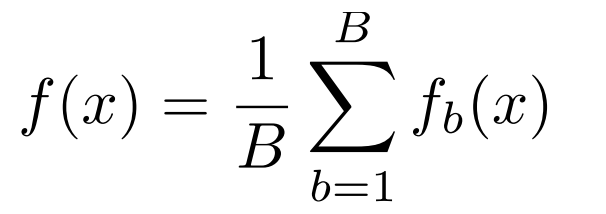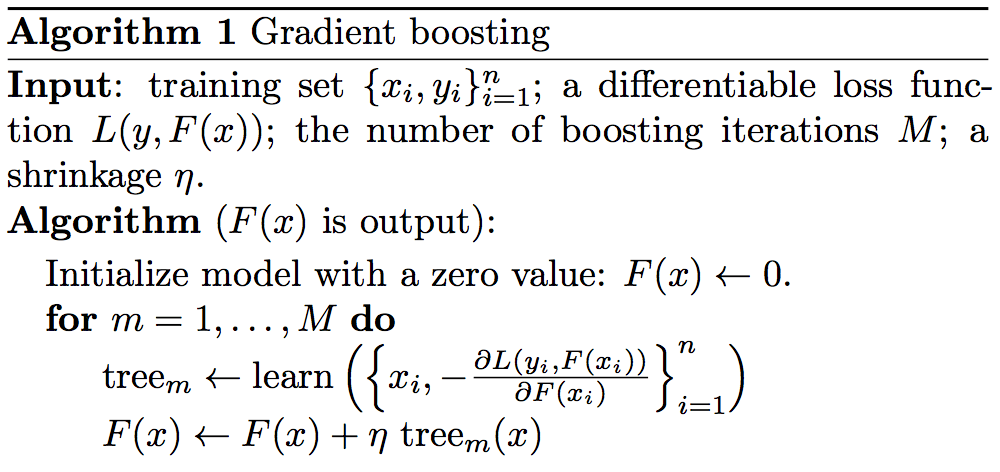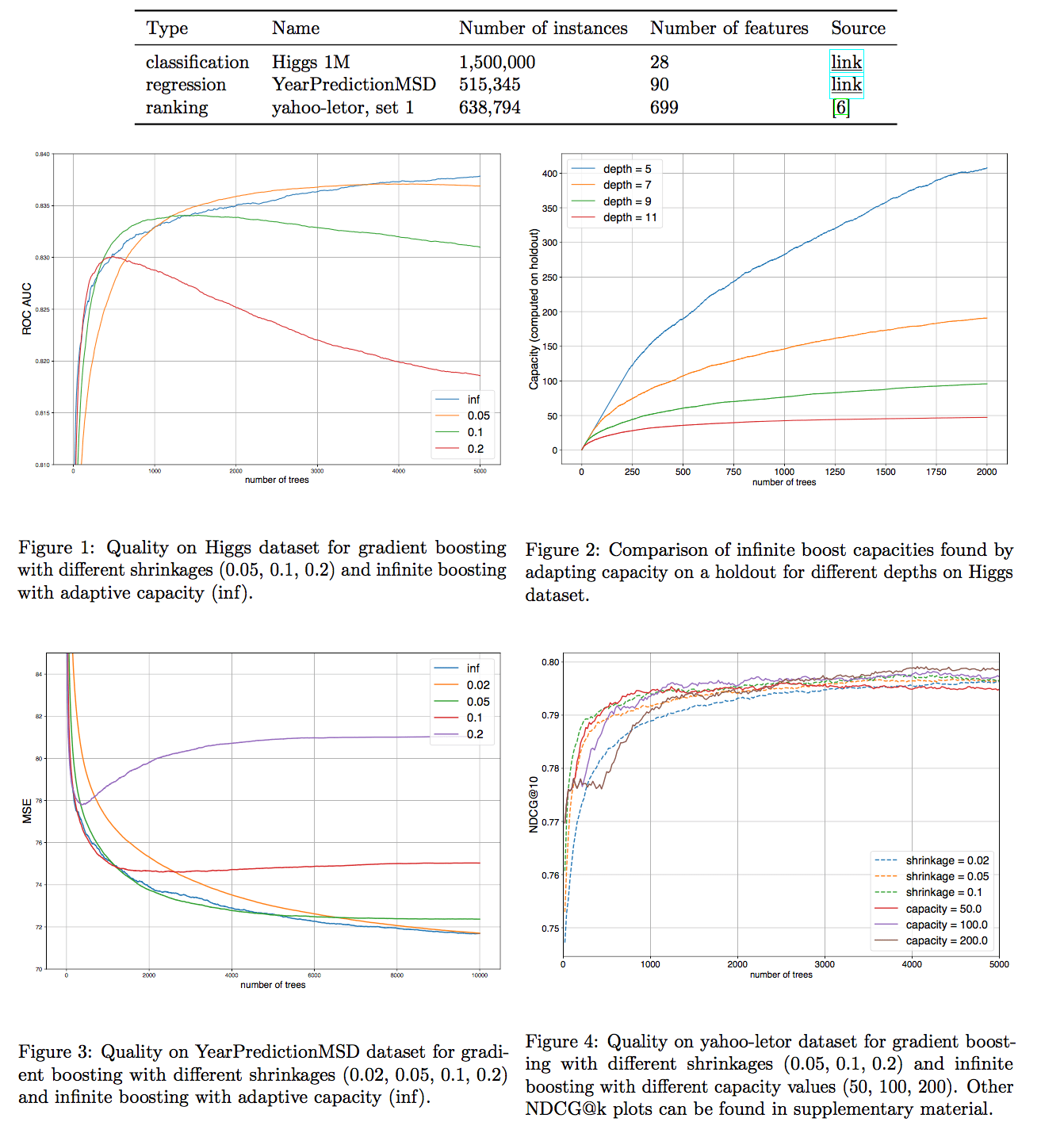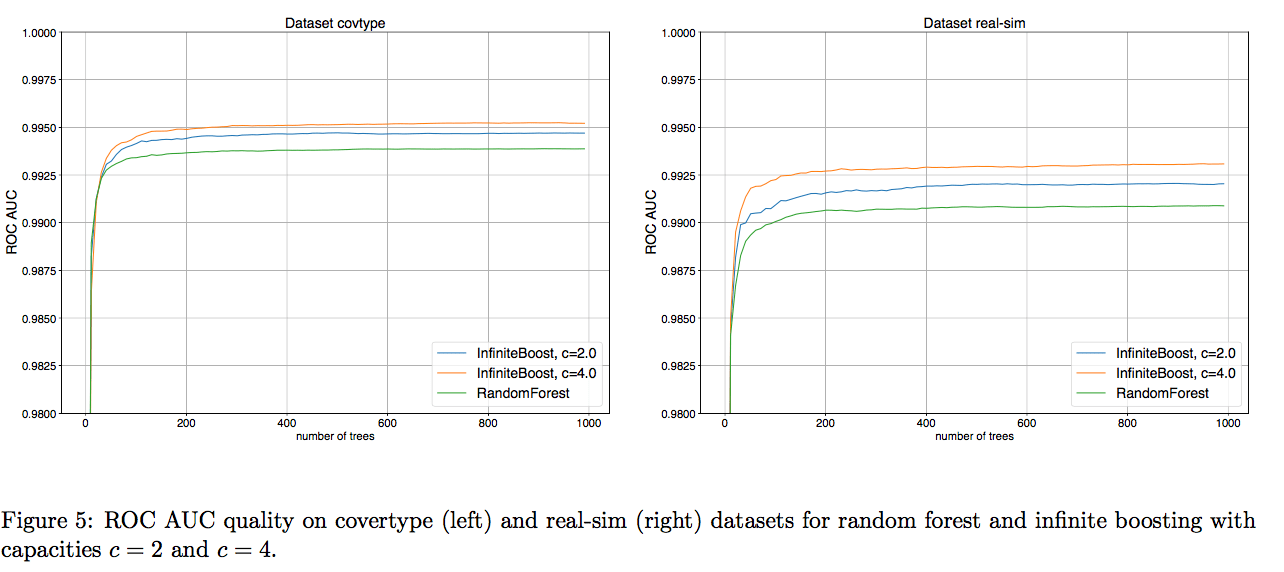# InfiniteBoosting – A Bagging-Boosting Hybrid Algorithm

InfiniteBoosting overcomes the disadvantages of both bagging and boosting, thus slightly improves the performance. Its most important aspect is its adaptability.

## Intro

Ensemble methods, due to their wide applications and fancy accuracy, are attractive since the day they were invented. In the family of ensemble methods, bagging and boosting algorithms share some common properties, but differ in training process. Generally speaking, bagging is good for reducing the variance, while boosting focuses on both bias and variance. However, it is well known that boosting tends to over-fit the dataset, thus leading to higher variance.

The effectiveness of ensemble methods is successfully proven by data competitions: 60% of winning solutions in Kaggle challenges used XGBoost (a popular boosting algorithm). An extremely strong statement from a winner of those competitions, Qingchen Wang just about sums it up: “I only use XGBoost”.

To combine the best properties of bagging and boosting, the author proposed a hybrid algorithm — InfiniteBoosting, which constructs an arbitrary large ensemble tree and trains every weak learner in a gradient boosting way. Due to its hybrid features, this algorithm builds a classifier reducing bias at each training step, while controlling the over-fitting at the same time.

## Bagging and Boosting

Let’s first take a look at bagging methods. Bagging is short for bootstrap aggregating, and the equation below demonstrates its principle: train many weak learners f_b(x) on bootstrapped dataset, and take the average to output the learning result. Here, bootstrap means randomly generating different data samples from the original dataset (roll n-faces dice n times).Following this idea, Random Forest algorithm is introduced naturally, since decision tree is a perfect candidate for weak learner. A single decision tree holds properties such as low bias and high variance. After aggregating a pack of trees, bias remains but variance goes down. We could achieve a constant bias that is as low as possible by constructing a large enough random forest.

Now let’s turn to boosting. The basic idea behind boosting is whether a weak learner could be modified to become better by focusing on improving its weakness. This is done by using the weak learning method multiple times to get a succession of hypotheses, each one refocusing on the examples that the previous ones found difficult and have misclassified. By doing so, boosting helps a weak learner grow into a strong one. The equation below shows that the final output of boosting is the weighted average (voting) of weak learners, where alpha_t is the weight calculated by considering the last iteration’s error.The image below shows the typical learning procedure of boosting algorithms. Each learner learns from the failures of the last learner, and outputs correct result by accumulating all learners’ output.## InfiniteBoosting

The goal of InfiniteBoosting is to build an infinite ensemble by boosting procedure. Unlike the Gradient Boosting method, InfiniteBoosting updates f(x) by averaging all weak learners at every iteration with weights alpha_t, then multiplied by a constant c called capacity, rather than a linear combination like in Gradient Boosting. To show the difference in detail, I quote the algorithm procedures in original paper:## Analysis

InfiniteBoosting algorithm introduces features from both bagging and boosting, using the same learning procedure as Gradient Boosting but updating the re-weighted output instead of accumulating mathematically. We could easily conclude that this algorithm is a random forest whose individual trees learn from the mistakes incurred by others; and alternatively, this is a modified version of Gradient Boosting, differing in the updating method.

The effectiveness of this algorithm is shown by the authors: it converges almost surely to the solution, as long as each tree’s output on the training data is bounded and continuous. Meanwhile, we should notice that the error of any machine learning algorithm is the sum of bias and variance. Bagging methods reduces the variance by making uncorrelations among learners to minimize the variance, but this causes bias to go up slightly. Boosting methods reduce bias by aggregating different models’ outputs, but causes variance to blow up. Thus, InfiniteBoosting, a hybrid of two methods, can never break through the limits of any of the two methods, and it may infinitely approach the results of bagging or boosting.

The obvious advantage is that InfiniteBoosting allows bagging or boosting to work on the tasks that they may not fit in the first place. In other words, this method is robust on different datasets or tasks. Given enough data, it could always achieve one of the best performance according to bagging and boosting.

However, to converge to a stationary point, it requires large enough datasets and longer training time. I guess this disadvantage will not impede too many applications, since data is everywhere nowadays, and computation resources are growing with time.

## Experiment ResultsFrom the result, we could conclude that InfiniteBossting shows similar, but slightly better results compared to Gradient Boost or Random Forest methods. The results also agree to the analysis performed in the last section.

## Conclusion

InfiniteBoosting overcomes the disadvantages of both bagging and boosting, thus slightly improves the performance. Its most important aspect is its adaptability. It extends the boundary of any single bagging or boosting, so more applications can taste the goodness of both bagging and boosting.

Author: Qintong Wu | Localized by Synced Global Team: Xiang Chen